All calculated data derived from FACE's functions can be used in FlowWorks' tools, in the same ways as any raw data channel. For example, calculated (virtual) data can be graphed and exported in a data table. This is achieved by first creating a calculated channel, and then appending a FACE function that is to be immediately applied to incoming data.

This topic provides a description and example for every predefined Function in F.A.C.E.

## Quick Reference Guide: Functions

The PDF found below provides a quick reference guide to all of the functions offered in F.A.C.E., as well as some notes on basic formulas and conditional statements.

To learn of each function in greater detail, explore the remainder of this article.

The Cumulative function is typically used to reduce the frequency of data points, by totaling data values within a proscribed time period. This is achieved without actually removing the number of points in the database. This function is useful when you would like to take a large step back and observe the bigger picture expressed by your data.

The Cumulative function works for any Channel in which the summation of data over a period of time makes sense, such as in the case of a 'Rainfall' Channel; as opposed to a measurement like 'Flow', which does not lend itself to summation.

Cumulative is not the same as Sum. Although the Sum function shares the similarity of totaling data points within a proscribed time period, Sum represents the interval total as a single data point, which reduces the total number of data points in your database; whereas the Cumulative Function does not reduce the number of data points.

### Example

We'll look at a Site at which the Rainfall Channel is reporting every 15 minutes. Let's say we want to see Rainfall data as a daily total, rather than how much rain fell in each of the day's 15-minute time slots - while this loses the granularity that allows us to look at a passing rainfall event, the daily interval is all we need.

Our original Rainfall data stream for one hour looks like this: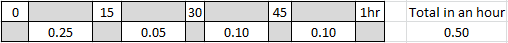The Cumulative function keeps the same data point frequency as the original, but adds the data in a running total. Instead of resetting the data value to zero at the conclusion of each 15-minute reporting period, the system adds that data value in a running total until we tell it to reset at our new 'reset frequency' (which in this example is 24 hours). With the Cumulative function, our new Calculated channel for the above period looks like this: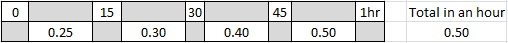As shown in the above image, instead of resetting the data value to zero at the end of each reporting period, new rainfall data is added to the previous interval data, until we reach the end of our new reset period (24 hours). Once the reset period has been reached, counting restarts back at zero and the process is repeated.

### A second example

The graph below shows a Cumulative Calculated Channel graphed along with the original data. This demonstrates a rain gauge that empties every 5 minutes and the daily resetting plot accumulated by the Calculated Daily_Rainfall Channel.This becomes more apparent when we zoom in to a level at which it is easier to see the rainfall activity of each day in a midnight through midnight accumulation / reset cycle.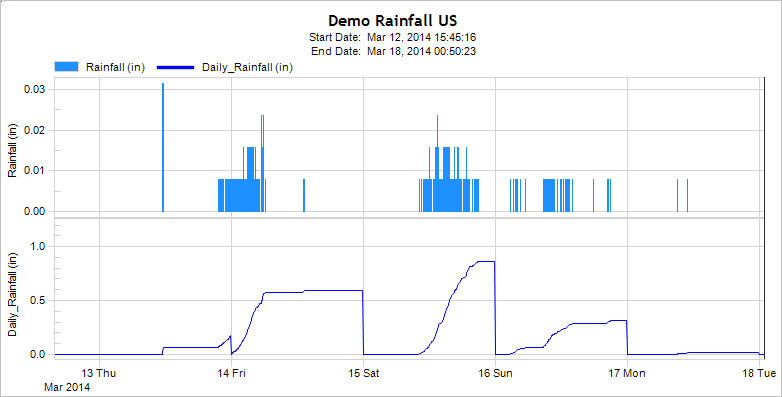The Delta function provides rate of change values that is observed over a specified period of time. When combined with FlowWorks' Alarm / Notification capabilities, the system can alert you to an undesired situation when the rate of change (or lack thereof) exceeds the parameters you set.

### Example

Let’s say we have an instrument measuring the depth of a catch basin. Knowing the absolute depth of the water in that basin at any given time is important; more importantly however is the ability to know the rate at which depth is increasing, to justify dispatching crew members.

Below is a table of absolute water depth levels in a basin: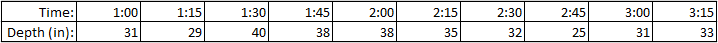The image below is a graph of the data in the above table: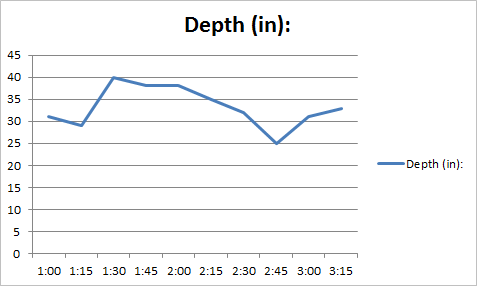By plotting the data, we can see that the depth is rapidly rising between 1:15 and 1:30. The increase in depth may not be of concern; however the rate of rise may indicate a systematic problem, should the rate of increase persist. Even though this graph shows that the rate of rise did not continue within this time period, it provides an indication of a potential problem in the long-run.

Using the Delta Function, we are able to subtract the depth value of a reading from the reading right after it. The result is a table of Delta values, or change in value observed over the specified time period.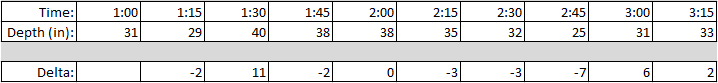F.A.C.E.'s Delta function compiles Delta values in a Calculated (also known as a Virtual) Channel, which is instantly available for use in Graphing and Reporting. Delta can also be used in conjunction with Alarm / Notification functions to alert you to those changes you consider to be significant.

The Delta Time function identifies data gaps. It does this by looking at the Date and Time Stamp that is recorded with each datapoint and calculates the amount of time (in seconds) between a single datapoint and the datapoint just previous to it.

### Example

If your data normally appears every five minutes, in a year that equals to 105,120 data points collected. If for some reason your Site did not capture data for a two hour period, that equates to 120 missing data points or 1/10th of a percent of the data. Finding that 'hole' in the data stream can be like searching for a 'needle in a haystack'. Working down a year's string of data, Delta Time identifies these gaps immediately. Used in conjunction with Alarm / Notification triggers, you can be alerted via email or SMS when data gaps grow too large, beyond a specified threshold. This function enables you to quickly identify when data stream problems took place, and serves as a starting point for answering the question for why data gaps occurred in the first place.

When a Calculated Delta Time Channel is added to data, any data gaps become easily visible. The table below demonstrates this. Notice that all data gaps are 300 seconds; except one, which is 7500 seconds long. This not only reveals that a data gap exists, but also where in the data it resides: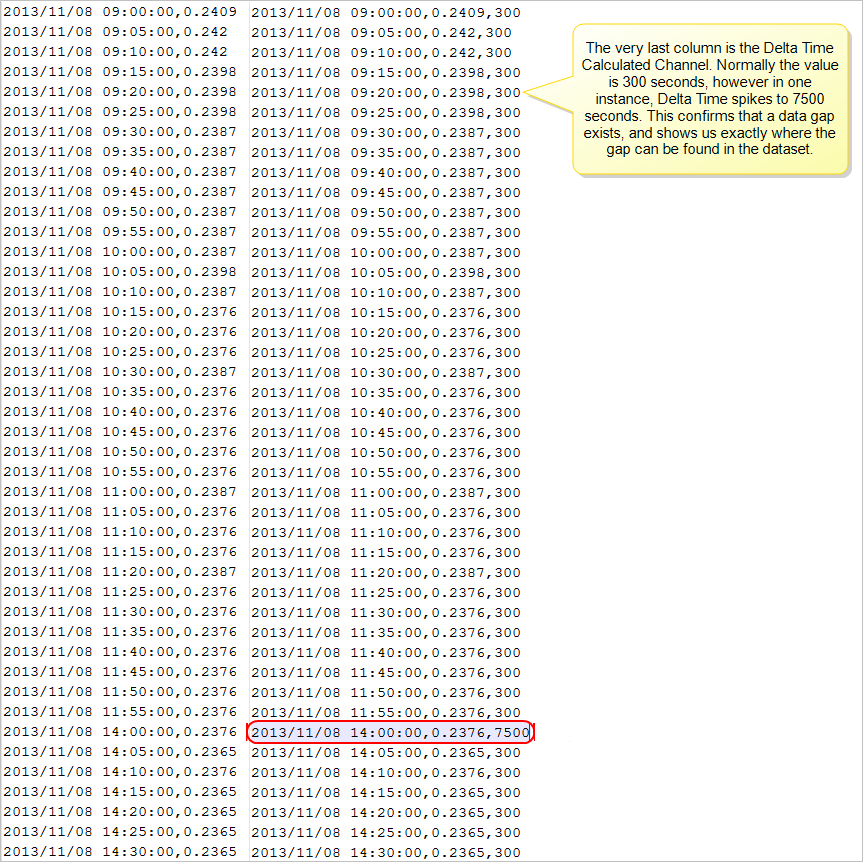Another way to view data gaps is to graph the Delta Time Channel, as shown below: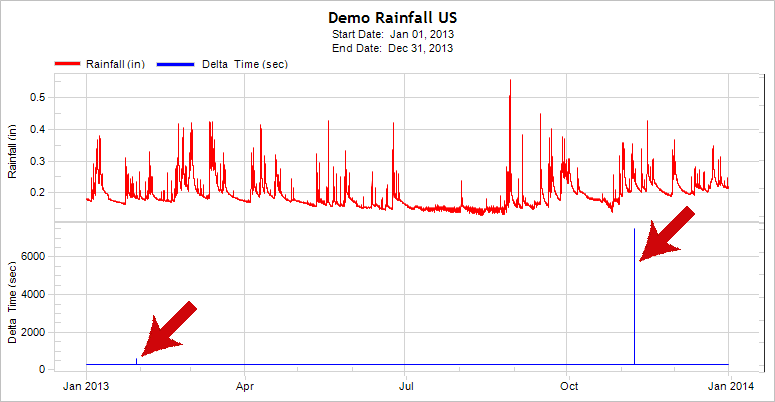The Time Series graph shows that there was one instance in which the data stream deviated from the expected 300 second mark. Identifying when and where these data gaps occurred enables you to troubleshoot the problem more effectively.

The Discrete function is used to create a fixed interval time series from a cumulative or totalizer source Channel. the optional rollover value can be used to set a maximum value on the totalizer, and any values that exceed this are added into the next interval.

### Example

Many of FlowWorks' advanced tools; including the Inflow and Infiltration (I & I) tool, and many functions in F.A.C.E.; require that data is in five minute intervals. Thus, a typical use case would be to turn a daily running total flow Channel into a five minute flow Channel.

The Interpolate function creates a fixed interval time series by linearly interpolating between the values of the source Channel. It is used when one wants to create data in smaller time intervals than that from the source channel, for non-rain gauge devices.

It is important to note that the Interpolate function does not alter the original data. Also, Interpolate is used to strictly create approximations of data points: think of this as taking two 15-minute interval data points, computing the difference between these, then dividing that difference into three slices, and adding it back to the first measurement. this approximates what likely happened during the interval between the two actual measurements. However, it could in fact be hiding a positive or negative spike of some kind. Without real-world measurements inside the 15-minute interval, Interpolate is used to compute a 'best guess' of what the data points in between are like - interpolated data are not an absolute reporting of measurements.

### Example

In this example, let's say you want to use the Interpolate function to create 5-minute interval data from 15-minute intervals for a Level Channel for use in the I & I tool.

The graph and table below shows seven days of a Site's real data, demonstrating that the data is in 15-minute intervals: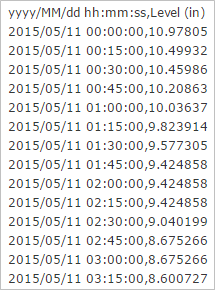By creating a Calculated Channel and configuring it with the Interpolate Function for an interval length of 5 minutes, F.A.C.E. will automatically break up the Level data and use mathematical estimations to fill in the gaps between each 15-minute interval.

By plotting the original Level channel and the new Calculated 5-minute data, you will get a graph that looks like this: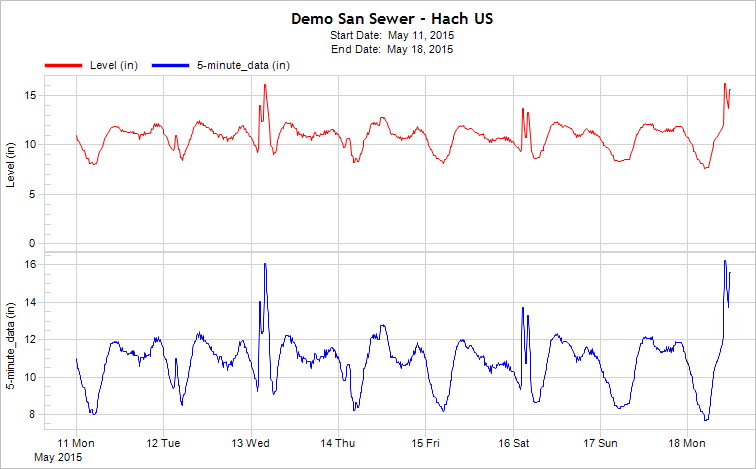Ignoring for a moment the scaling differences, you can see that while we have created 5-minute data (the blue line), we have not altered the fidelity of the resulting plot. This can be further demonstrated by exporting the data which created these plots: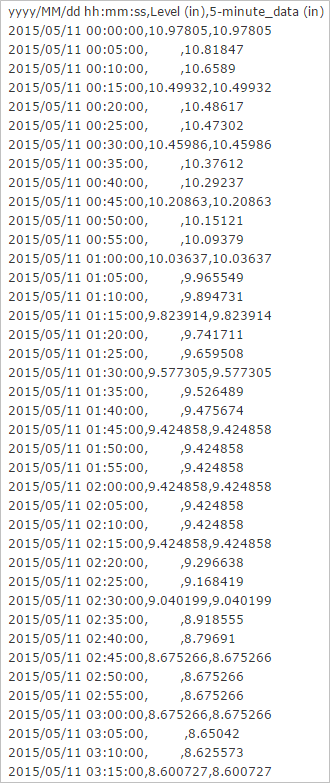As seen in the table above, the Interpolate Function was able to successfully approximate 5-minute interval data values from 15-minute interval data. You can now use the new Calculated Channel in lieu of the original Channel for I & I analysis.

The Manning Flow Function calculates flow based on the water level, pipe size, slope, and roughness coefficient using the Manning flow equation for circular pipes. Results for imperial units are in cubic feet/second, and cubic meters/second for metric units.

### Example

A typical use would be for quality control of recorded flow data, by comparing the flow data against the computed Manning flow.

The Manning Velocity Function calculates velocity based on the level in a circular pipe for a given pipe size, slope and roughness coefficient, using the Manning Velocity Equation. Results for imperial units are in feet/second, and meters/second for metric units.

### Example

A typical use would be for quality control of recorded velocity data by comparing recorded velocity against the computed Manning velocity.

The Maximum function allows you to quickly identify the maximum value of any given data Channel, within a given range.

### Example

Infrastructure is akin to a living, dynamic organism; engineers face the continuing challenge of maintaining its equilibrium. In doing so, knowing the largest (and smallest) data values of a particular variable can provide insights paramount for quality control and safety; such as in the case of comparing a neighborhood sanitary sewer's water flow capacities to the maximum flow reached during a rainy month. The Maximum value can tell us when - and how often - our infrastructure is reaching its threshold.

Just as with any other function, the Maximum is configured in a Calculated (Virtual) Channel. Once set up, this Channel can be graphed parallel to other channels in your database, for comparison and analysis. The graph below shows the maximum flow for every 5-minute period per week, within the three-month span: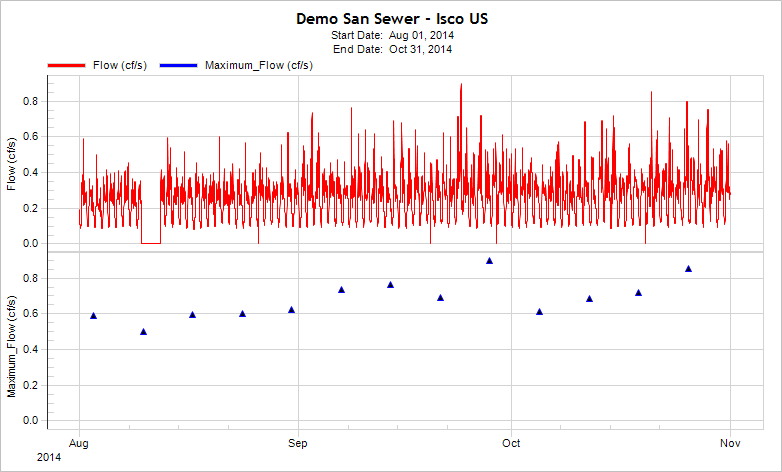The Median function creates a fixed interval time series by computing the median of all the values within the specified interval.

### Example

An example use for this function would be to find the median daily temperature by choosing the raw temperature Channel with smaller time step and specifying a daily interval.

The Minimum function allows you to quickly identify the minimum value of any given data Channel, within a given range.

### Example

For any community in the world, life is dependent on the existence of water. Engineers face the continuing challenge of ensuring our water is not only kept safe, but also in abundance. In meeting this challenge, knowing the smallest (and largest) data values of a particular variable can provide insights paramount for quality control and safety; such as in the case of knowing the lowest depth of a water reservoir, that is depended by a town for its water supply. Minimum values can tell us when - and how often - availability of our water is reaching its limits.

Just as with any other function, Minimum is configured in a Calculated (Virtual) Channel. Once set up, this Channel can be graphed parallel to other channels in your database, for comparison and analysis.

The Pump Station Calculator is intended for use across a wide range of pump station configurations. The FlowWorks Pump Station Calculator will turn mounds of under-utilized data from pump station dataloggers and SCADA systems into valuable pump station performance information, such as efficiency and threshold information.

The Pump Time-Weighted Average function creates a weighted average based on the time between zero valued results (i.e. pump off) that begin and end a succession of non-zero valued results (i.e. pump on). This function is primarily used with pump station start & stop signals in order to compute the average time of pump runs. Particularly, this function will provide insight for pump operators to determine if their pumps are overworked.

The Repeat function is used to produce a repeating signal of data. It fills the selected range with repeated sets of data from the specified source Channel in a specified date range.

### Example

An example use case of the Repeat function would be to create a dry-weather flow pattern, which could be used to compare flow data collected during a rainfall event.

Another useful application would be to use this function to identify relative growth within a catchment if several years of flow monitoring data is available.

The Rolling Average function is used to analyze trends in a data set, by creating a series of averages of any subset of the data. It can be Calculated for any period of time and is extremely valuable for forecasting long term-trends.

### Example

Typical uses include calculation of duration-based rainfall statistics for alarming - for example, calculating the average rainfall in a 5-minute reporting period for a rolling week window. This allows for a more generalized picture of the data set, to help better determine long-term trends.

Once you have created a Calculated Channel for Rainfall Rolling Average, you can graph it alongside the raw Rainfall Channel for comparison. One can begin to see how the average rainfall in any 5-minute time span is affected by the events depicted in the upper plot:The data in any Channel can be exported as a .CSV file, compatible with Excel. The output table is can be used to generate graphs and reports: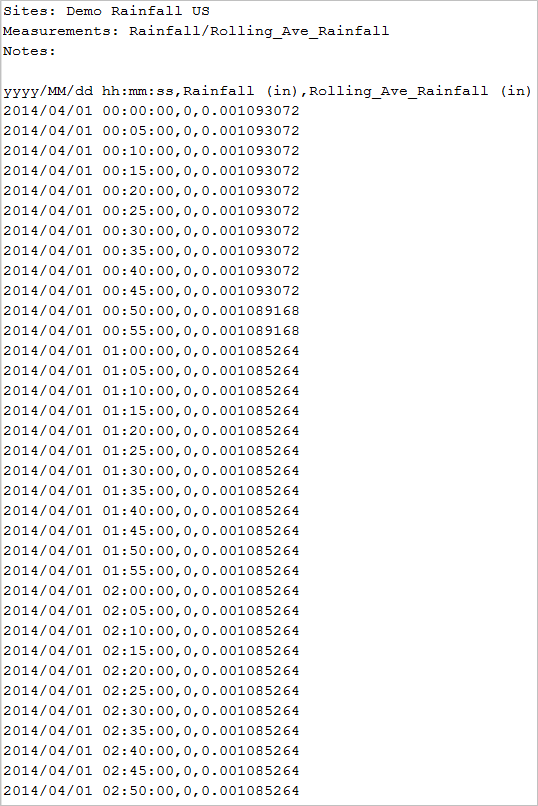This saves the user significant time in having to manually apply a SUM formula in Excel. The resultant table turns out to be exactly the same: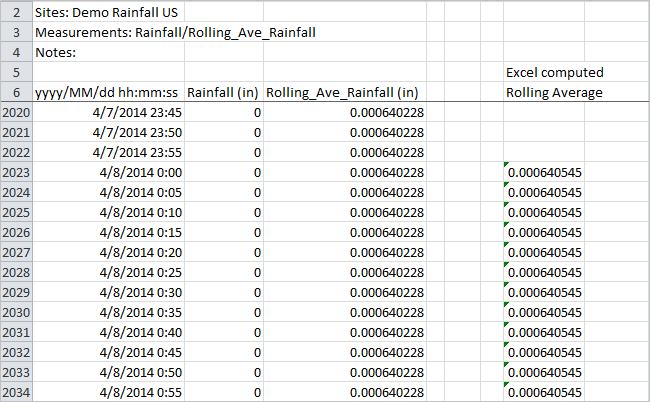Rolling Sum is the total of a number series, which updates each time a new number is added to the series, within a given time period.

### Example

While those of us in the State of Washington like to perpetuate the rumor that it rains all the time here, the data tells us that most of our rainfall events are more like drizzles - it is only on occasion that we get a string of heavy precipitation. This can be concerning because much of the state sits on a layer of glacial clay. When combined with a hilly terrain, soaked soil sitting on that clay layer can (and does) raise the potential for slides ranging from a nuisance to catastrophic. If given a few days to dry out, everything goes back to relatively safe; but in the meantime, it is useful that we know what the Total Rainfall has been in any reporting period over the last week (seven days), to determine wetness and volatility of the clay layer.

Once you have created a Calculated Channel for Rainfall Rolling Sum, you can graph it alongside the raw Rainfall Channel for comparison.

The sum values in this Channel can be exported as a .CSV (comma separated values, a.k.a. comma delimited) file, compatible with Excel. The output table is completely graph-able and reportable. This can save you time in having to manually apply a SUM formula in Excel.

the Shift function is used when you need to move a segment of data backward or forward in time.

### Example

FlowWorks' recommendation is that data be kept in Standard Time throughout the year. Yet when a crew looks at the daily cycles of levels in a particular conduit into which they must enter for maintenance work, they want to select the 'low level' period of the work day. If you are not only keeping, but also displaying your data in Standard Time, your crew must do the mental adjustment between the graphed data they receive from FlowWorks and their wristwatches.

With Shift you can leave your data in Standard Time while displaying it in Daylight Time by shifting the data forward one hour. There is no gap in the data being recorded and no overwrite when the local time returns to Standard. Yet when your users look at or graph your data, they see it represented in local time.

For a further discussion of the inherent problem involved with storing data with Standard/Daylight time stamps, see topic, "Field Equipment: Considerations".

The Step Function takes a value you have computed and places it into each of the new data points.

There are two ways to describe what happened in the interval between two actual measurements. the first method is interpolation and works well for measurements like Flow and Velocity that has a continuously varying value. The other is Step and works for data like that from rain gauges, which provides more 'chunky' data.

In the first instance (interpolation), you can approximate any number of points between two measurements by computing the difference in the measurements, dividing that difference by the number of 'new' data points you want to wedge between two measurements. Then starting with the first datapoint, add one of the 'difference pieces' to that measurement. Repeating as you move across the time line you should end up at the second actual measurement with a matching datapoint value. FACE offers an easy way to accomplish this with the Interpolation function.

In the second instance (Step), because rain gauges typically log data in 'dumps', using the Interpolate function creates rain that did not exist in reality. For this we can use Step to take a previously computed value and place it into each of the new data points.

### Example

The Step Function can be used to break 'dumped' data such as Rainfall Gauge data, into 5-minute intervals. This can be useful for analyzing the data with FlowWorks' I & I Estimator; it also enables you to break-up your data for more targeted analysis.

Shown in the images below, we have graphed the data for the dates January 5th and 6th 2014 and then used the Tool / Export Data options to export the data. In the table you can see how when we have 0.01 inches of rain measured at 20:45 or 1/5/2014, we divided that into three pieces each having a value of 0.0033… and then put them into data points at 20:30, 20:35, and 20:40.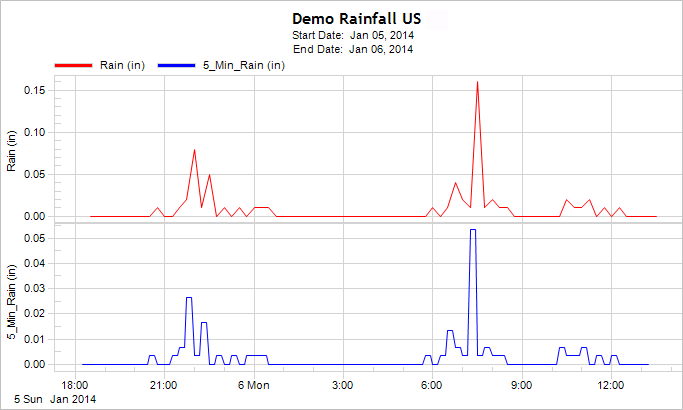This function is not the same as the Cumulative Function. Although it shares the similarity of totaling data points within a proscribed time period, Cumulative does not reduce the total number of data points. It simply displays totals within a time interval. Whereas the Sum function does reduce the number data points.

### Example

To use the Sum Function you need as a source of data, a Channel with a data stream like “Rainfall.” That is to say a Channel in which the summation of the data over a period of time makes sense; as opposed to a measurement like “Flow” which does not lend itself to summation.

We’ll look at a Site at which the Rainfall Channel is reporting every 15 minutes. And for our example we will say that we’d like to see that Rainfall as a daily total rather than how much rain fell in each of the day’s 15-minute time slots. While this loses the granularity which lets us look at a passing rainfall event, it may be the interval at which some reporting agency would like us to provide data.

Thus, our original rainfall data stream for an hour would look like:There are two functions in the FlowWorks Advanced Calculation Engine which can work from frequently reported data and output the results as is the data was collected at longer intervals – the Cumulative function and the Sum function. The differences are in the reporting frequency. The Cumulative function keeps the same datapoint frequency as the original, but instead of resetting the data value to zero at the conclusion of each reporting period, it adds that data value in a running total until we tell it to reset at our new “reset frequency”. Thus with the Cumulative function, our new Calculated channel for the above period would look like: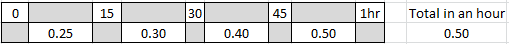where instead of resetting the data value to zero at the end of each reporting period, we add the new rainfall to that which we have already seen until we reach the end of our new reset period. Thus as the next hour’s collection begins, we are again at zero and repeat this process.

The Sum function also adds the data values found in the original data stream and resets at our newly proscribed reset period, but it also skips reporting at the original source channel’s data collection frequency and reports only at the reset period we have set. Hence for the same input data, the Sum function’s reporting would look like: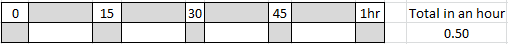The graph below shows a Sum Channel graphed along with the original Rainfall channel, demonstrating both the summation of the data and the adjustment in the datapoint frequency: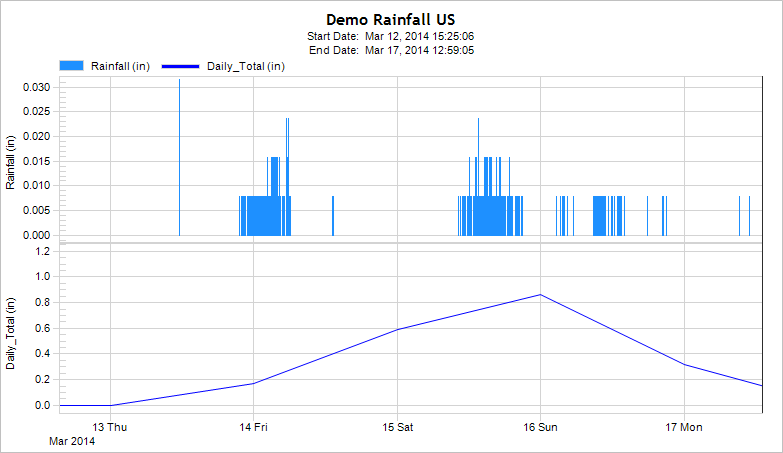The Time-Weighted Average Function creates a fixed interval time series of the average of the values within a specified time interval from the source Channel. The function computes the mean of all the values in specified interval based on the amount of time each value was present in the interval. Results are created at the end of each interval.

### Example

An example use of this function would be to compute the daily average flow from a 5-minute flow channel, and setting the interval to 1440 minutes (24 hours).